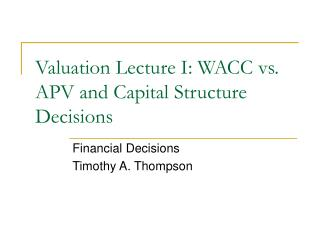DownloadDownload PresentationValuation Lecture I: WACC vs. APV and Capital Structure Decisions

# Valuation Lecture I: WACC vs. APV and Capital Structure Decisions

Télécharger la présentation## Valuation Lecture I: WACC vs. APV and Capital Structure Decisions

- - - - - - - - - - - - - - - - - - - - - - - - - - - E N D - - - - - - - - - - - - - - - - - - - - - - - - - - -
##### Presentation Transcript

1. Valuation Lecture I: WACC vs. APV and Capital Structure Decisions Financial Decisions Timothy A. Thompson

2. Market value balance sheet Market value assets Market value claims Excess Cash Total enterprise value Total enterprise value Debt Net debt Value of Projects Value of Projects Equity Equity Debt tax shields Debt tax shields

3. Asset or claim C = excess cash A = value of projects (unlevered) D = mkt value debt E = mkt value equity TS = debt tax shields Net debt = D’ = D – C TEV = D – C + E Required return, beta rc, βc ra, βa,reu,βeu rd,βd reL,βeL rTS,βTS Notation

4. Balance sheet mathematics • Logic: • LHS and RHS of B/S must be equal • Assume that TS refers to the present value of tax shields net of costs of financial distress

5. Calculating the equity value of the firm • Equity method • Present value of all future cash flows to equity • Discounted at the required return on the levered equity of the firm, reL, (based on levered equity beta, βeL) • reL is greatly affected by differences in leverage • Method buries future net debt payments into the equity cash flow • Valuation by components • E = TEV + C – D

6. Valuation by components method • Value the total enterprise value of the firm by discounting all the operating asset cash flows • Discounted at either • WACC (using the WACC method) • Or at • The unlevered cost of equity reU (APV method) • What is the difference between WACC and reU? • TAX SHIELDS • Equity = Total enterprise value + Excess Cash – Market Value of Financing Obligations

7. Value of investment is the present value of the investment’s cash flows (DCF) • Cash flows are measured as free cash flows from operations • From operations means no financing-related cash flows are included • Free cash flows means after expected new investments • When financial structure matters either • Discount operating free cash flow at WACC • WACC incorporates tax benefits of debt into the valuation by reducing the discount rate relative to reU , WACC method • Discount operating free cash flow at reU (gives VU) • Then add the present value of tax benefits of debt financing explicitly (VL = VU + PVTS), APV method

8. What about costs of financial distress? • Theoretically, whatever costs of financial distress (COFD) have not been subtracted off in the operating cash flows should be subtracted from either method (WACC or APV) • Often difficult to estimate COFD • Good to develop intuition about what COFD are • Understand what business likely to suffer from large/small COFD

9. Capital Structure Tradeoff theory of the capital structure Value of the firm is a function of capital structure (in particular, the debt/value ratio) As firm levers up (from zero leverage, holding investments fixed) value increases due to PVTS (and perhaps for other reasons) As firm levers up, the value decreases due to COFD Want to find the happy medium!

10. Tradeoff Theory of the Capital Structure Maximum value of firm Costs of financial distress PV of interest tax shields Market Value of The Firm Value of levered firm Value of unlevered firm Optimal amount of debt Debt

11. How do you calculate PVTS? Simple model, Fin II • For example in the 40% debt scenario • Assume that the level of debt in the scenario is perpetual debt

12. How do you calculate COFD? • Difficult • COFD is the present value of all future expected costs associated with financial distress • If you did a method in Fin II, you can go ahead and attempt it • You would need inputs that are not in the case, and you would have to look them up • If you didn’t learn a method in Fin II • You can try to figure out a good guess from Chapter 17/18 of BM

13. Structure of COFD • COFD is a function of • The probability of financial distress • The cost of financial distress conditional on the firm becoming financially • COFD could be small even if distress is likely, if the firm would not likely incur large costs in distress • However, if we are pretty certain that the probability of distress is very small, then the above “product” is likely to be very small and PVTS is likely to exceed COFD

14. Bond Ratings • Investment Grade Ratings • AAA, AA, A, BBB (S&P) • Junk Bond Ratings • BB, B, CCC, etc. • If proforma bond rating (based on a certain capital structure) is high investment grade • AAA or AA, maybe even high A • Then probability of subsequent bankruptcy is very low (see BM) – these would very likely be safe capital structures